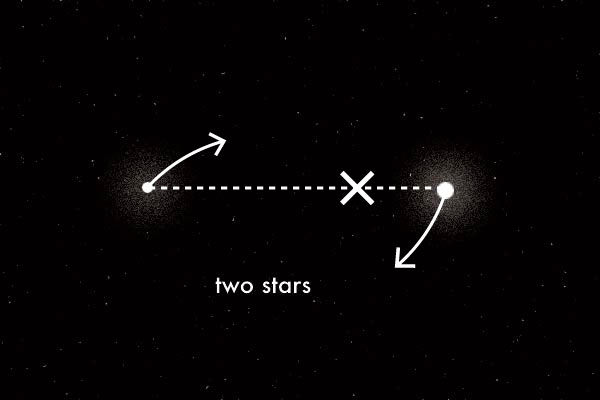# Star WaltzTwo stars orbit their common center of mass as shown in the diagram below. The masses of the two stars are $3M$ and $M$. The distance between the stars is $d$. Determine the period of orbit for the star of mass $3M$.

Note: This problem appeared on the 2009 F = ma exam.

×

Problem Loading...

Note Loading...

Set Loading...